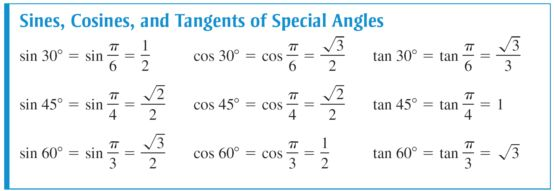# 国际课程提前学，A-Level数学之三角函数2：基础三角函数

Sin 正弦，cos 余弦，tan 正切，是三个最常用的三角函数。

The simplest definitions of the trigonometrical functions are given in terms of the ratios of the sides of a right-angled triangle, for values of the angle θ between 0° and 90°.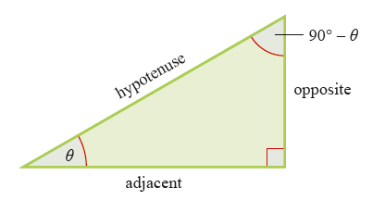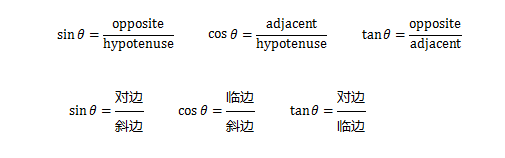Example1

Evaluating Trigonometric Functions

Use the triangle to find the values of .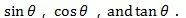Solution:

By the Pythagorean Theorem,

(hyp)2 = (opp)2 + (adj)2, it follows that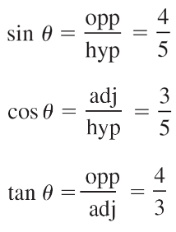Example2

Find the three trigonometric ratios  of  the angles 30°and 60°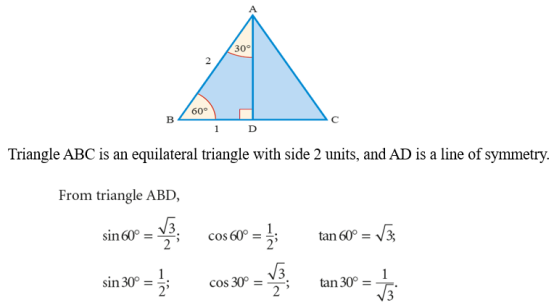Exercise1

Find the three trigonometric ratios of the angle 45°

The triangle PQR is a right-angled isosceles triangle with equal sides of length 1 unit.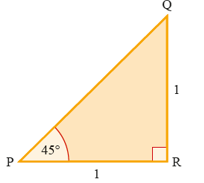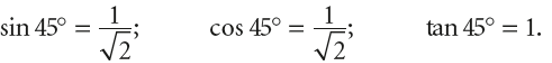Trigonometric function of special angles